Next: 6.2 Plasma Etching Up: 6. Applications Previous: 6. Applications

# 6.1 Chemical Vapor Deposition

In , a simple model, which is able to describe low pressure CVD and PVD processes, was presented. It assumes ballistic particle transport at feature-scale and considers only a single particle species. Particles remain sticking on the surface according to a given sticking probability. Otherwise, the particles are reemitted from the surface, and their directions follow the Knudsen cosine law (2.18). For non-linear surface reactions, a dependence of the local sticking probability on the incident flux was derived (2.32), leading to a recursive problem.

First, in order to compare the results and to verify the simulator, some two-dimensional low pressure CVD simulations, initially published in  are reproduced. The initial structure is a quadratic trench. The directional distribution of arriving particles is also assumed to obey a cosine distribution (2.5). The results for various sticking coefficients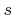and reaction orders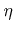are shown in Figure 6.1. The value ofcorresponds, in the case of non-linear surface reaction (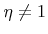), to the sticking probability on a plane surface. The results are in good agreement with those given in .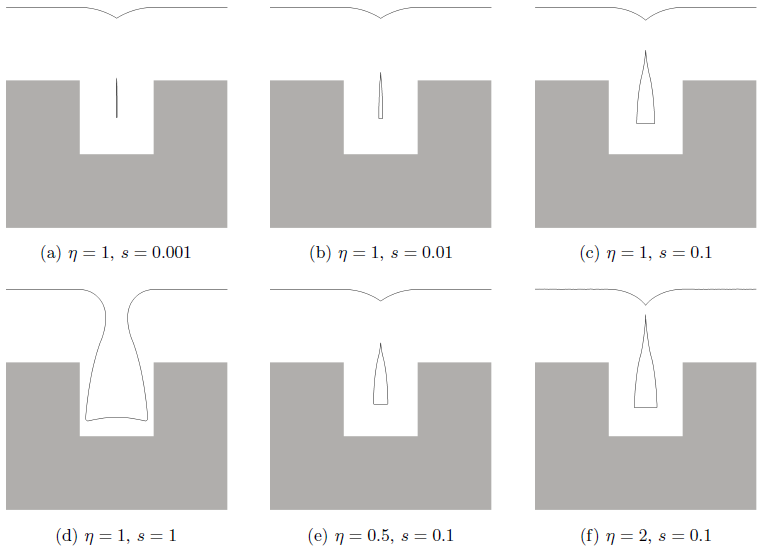The well behaving scaling laws of all the numerical techniques allow the simulation of large three-dimensional structures. In order to demonstrate the capabilities of the simulator, a deposition process is simulated for a large initial structure as shown in Figure 6.2. Its lateral extensions are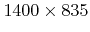grid spacings. The final profiles after deposition of a 15 and a 30 grid spacings thick layer are shown in Figure 6.3 and Figure 6.4, respectively. The deposition process was modeled using the parameters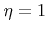and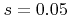.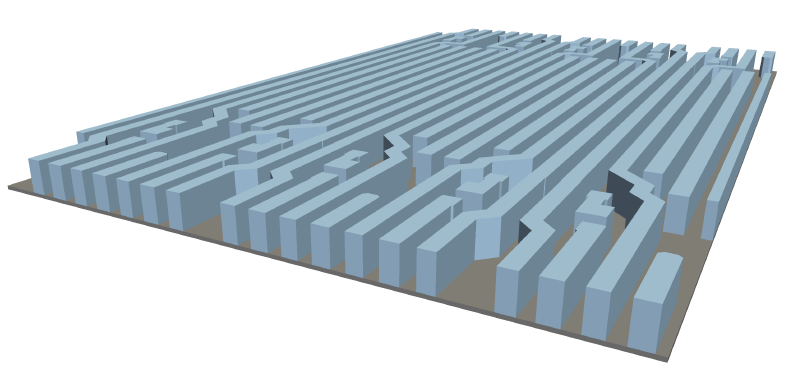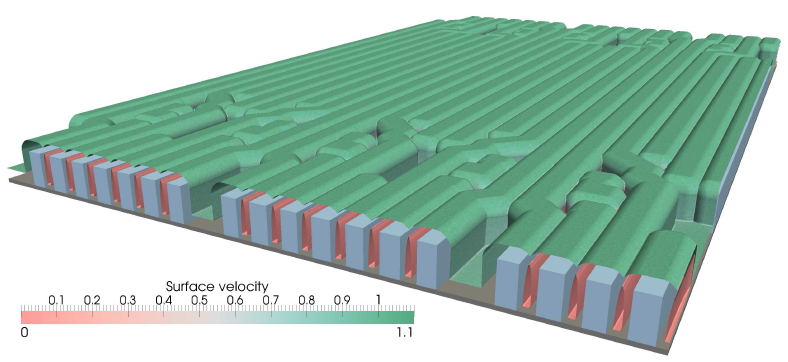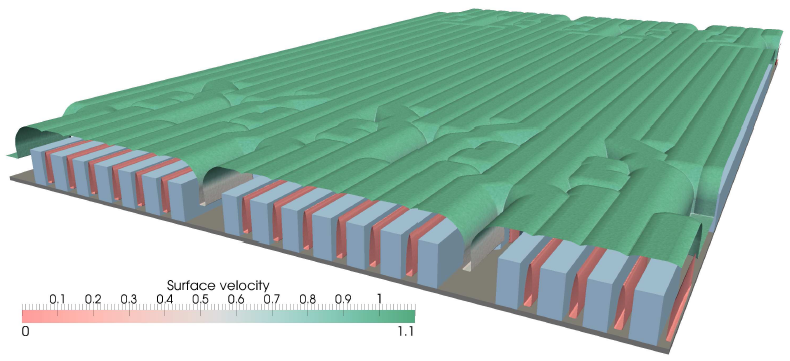For the simulation 1168 million particles are simulated at every time step in order to calculate the local deposition rates. Every time a particle hits the surface, a new particle is launched, as long as its new weight factor is larger than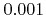. The simulation was carried out in parallel on 24 cores of AMD Opteron 8435 processors (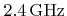). The average calculation time for a time step is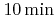. In total, 600 time steps are necessary for the entire simulation, when using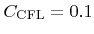for the CFL criterion.Next: 6.2 Plasma Etching Up: 6. Applications Previous: 6. Applications

Otmar Ertl: Numerical Methods for Topography Simulation